# Python集合是什么及怎么创建

### 集合（Set）

```thisset = {"apple", "banana", "cherry"}
print(thisset)```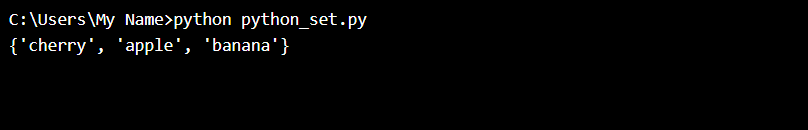### 访问项目

```thisset = {"apple", "banana", "cherry"}
for x in thisset:
print(x)```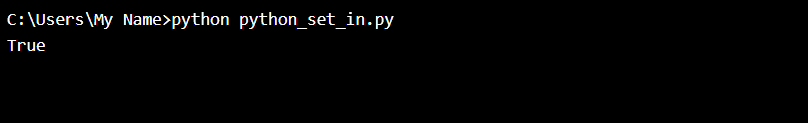```thisset = {"apple", "banana", "cherry"}
print("banana" in thisset)```

### 添加项目

```thisset = {"apple", "banana", "cherry"}
print(thisset)``````thisset = {"apple", "banana", "cherry"}
thisset.update(["orange", "mango", "grapes"])
print(thisset)```### 获取 Set 的长度

```thisset = {"apple", "banana", "cherry"}
print(len(thisset))```### 删除项目

```thisset = {"apple", "banana", "cherry"}
thisset.remove("banana")
print(thisset)```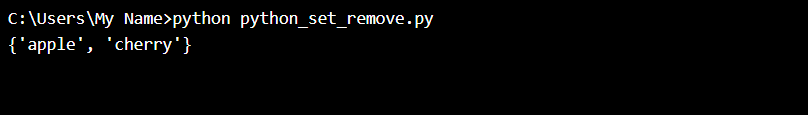```thisset = {"apple", "banana", "cherry"}
print(thisset)```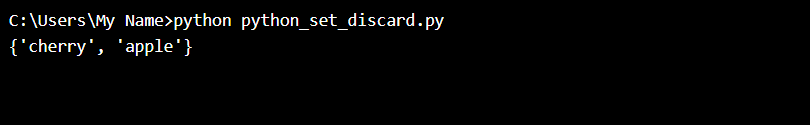pop() 方法的返回值是被删除的项目。

```thisset = {"apple", "banana", "cherry"}
x = thisset.pop()
print(x)
print(thisset)```clear() 方法清空集合：

```thisset = {"apple", "banana", "cherry"}
thisset.clear()
print(thisset)```del 彻底删除集合：

```thisset = {"apple", "banana", "cherry"}
del thisset
print(thisset)```### 合并两个集合

union() 方法返回一个新集合，其中包含两个集合中的所有项目：

```set1 = {"a", "b" , "c"}
set2 = {1, 2, 3}
set3 = set1.union(set2)
print(set3)```update() 方法将 set2 中的项目插入 set1 中：

```set1 = {"a", "b" , "c"}
set2 = {1, 2, 3}
set1.update(set2)
print(set1)```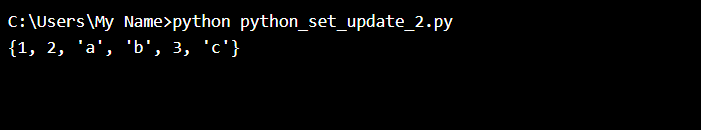### set() 构造函数

```thisset = set(("apple", "banana", "cherry")) # 请留意这个双括号
print(thisset)```### Set 方法

Python 拥有一套能够在集合（set）上使用的内建方法。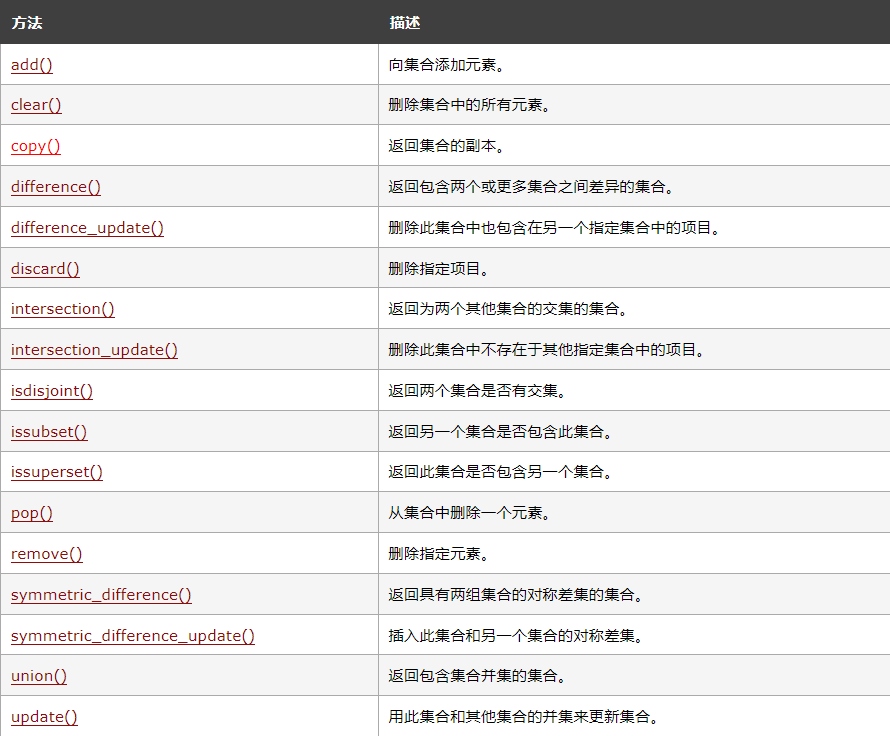### 评论